NVIDIA 2D Image And Signal Performance Primitives (NPP)  Version 11.3.0.*
Image Proximity

Primitives for computing the proximity measure between a source image and a template image. More...

## Modules

SqrDistanceFull_Norm
Primitives for computing the normalized Euclidean distance between two images with full mode.

SqrDistanceSame_Norm
Primitives for computing the normalized Euclidean distance between two images with same mode.

SqrDistanceValid_Norm
Primitives for computing the normalized Euclidean distance between two images with valid mode.

CrossCorrFull_Norm
Primitives for computing the normalized cross correlation between two images with full mode.

CrossCorrSame_Norm
Primitives for computing the normalized cross correlation between two images with same mode.

CrossCorrValid_Norm
Primitives for computing the normalized cross correlation between two images with valid mode.

CrossCorrValid
Primitives for computing the cross correlation between two images with valid mode.

CrossCorrFull_NormLevel
Primitives for computing the normalized cross correlation coefficient between two images with full mode.

CrossCorrSame_NormLevel
Primitives for computing the normalized cross correlation coefficient between two images with same mode.

CrossCorrValid_NormLevel
Primitives for computing the normalized cross correlation coefficient between two images with valid mode.

## Detailed Description

Primitives for computing the proximity measure between a source image and a template image.

# General Introduction

There are basically two approaches to compute the proximity measure for template matching, Euclidean distance and the cross correlation.

1. Euclidean distance computes the sum of the squared distance (SSD) between the corresponding pixels of the source image and the template image. The smaller the distance is, the more similar the source image and the template image is around the pixel. The anchor of the template image is used during the computations, which always lies in the gemotric center of the image. Given a source image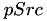(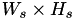) and a template image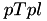(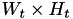), the Euclidean distance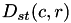between two images at pixel in row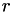and column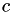is computed as (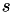stands for source image and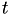for template image for short):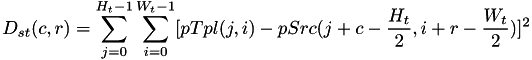2. Cross correlation computes the sum of the product between the corresponding pixels of the source image and the template image. The cross correlation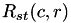is calculated as: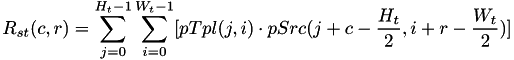The larger the cross correlation value is, the more similar the source image and the template image is around the pixel.
3. The cross correlationis affected by the brightness of the images which may vary due to the lighting and exposure conditions. Therefore, NPP computes the cross correlation coefficient to circumvent this dependence. This is typically done at every step by subtracting the mean from every pixel value, i.e.,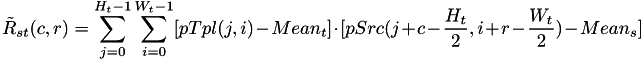NPP computes the normalized values of Euclidean distance, cross correlation and the cross correlation coefficient.

1. The normalized Euclidean distance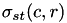is defined as: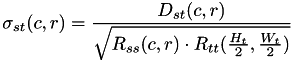2. The normalized cross correlation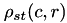is defined as: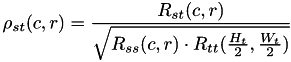The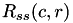and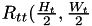denote the auto correlation of the source image and the template image individually. They are defined as: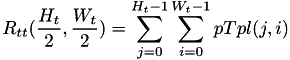3. Similarly, the normalized cross correlation coefficient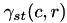is calculated as:The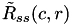and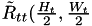are defined as: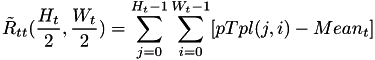# Categorizations

The Euclidean distance and the cross correlation are categorized into three types, full, same, and valid.

1. Full mode indicates that the anchor of the template image starts from the outside of the source image, assuming the out-of-boundary pixels are zeor-padded. The size of the destination image is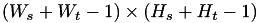.
2. Same mode means that the anchor of the template image starts from the top left pixel of the source image. All the out-of-boundary pixels are also zero-padded. The size of the destination image is the same as the source one, i.e.,.
3. Valid mode indicates that there are no out-of-boudnary readings from the source image. The anchor of the template image starts from the inside of the source image. The size of the destination image is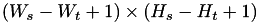.